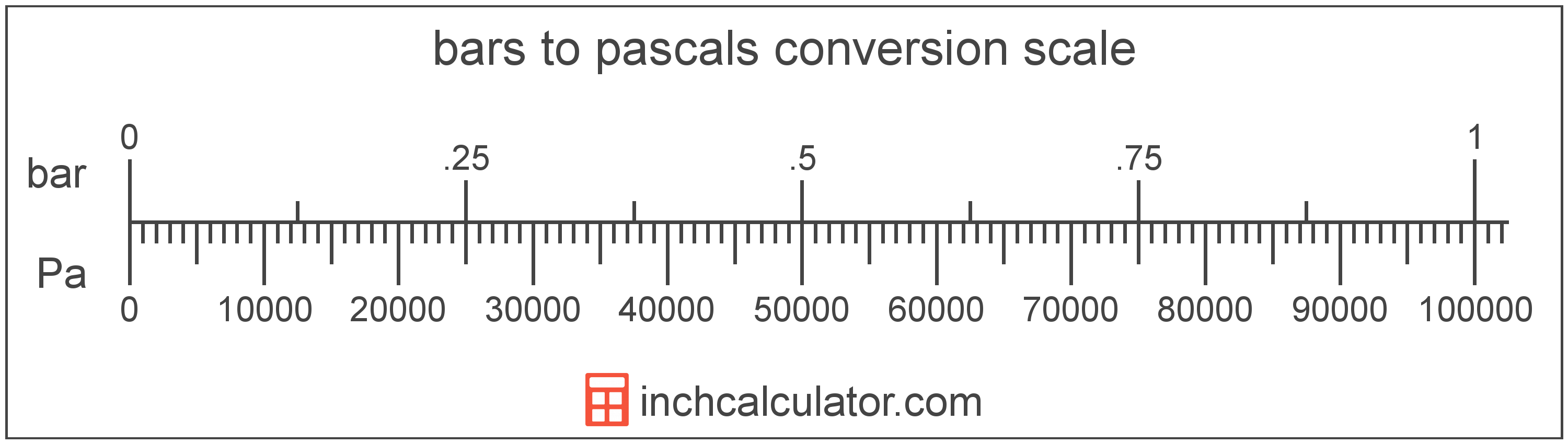# Pascals to Bars Converter

Enter the pressure in pascals below to get the value converted to bars.

Results in Bars:1 Pa = 1.0E-5 bar
Hint: use a scientific notation calculator to convert E notation to decimal

Do you want to convert bars to pascals?

## How to Convert Pascals to Bars

To convert a measurement in pascals to a measurement in bars, divide the pressure by the following conversion ratio: 100,000 pascals/bar.

Since one bar is equal to 100,000 pascals, you can use this simple formula to convert:

bars = pascals ÷ 100,000

The pressure in bars is equal to the pressure in pascals divided by 100,000.

For example, here's how to convert 50,000 pascals to bars using the formula above.
bars = (50,000 Pa ÷ 100,000) = 0.5 bar## What Is a Pascal?

The pascal is defined as the pressure of one newton per square meter.

The pascal is the SI derived unit for pressure in the metric system. Pascals can be abbreviated as Pa; for example, 1 pascal can be written as 1 Pa.

Pascals can be expressed using the formula:
1 Pa = 1 N / m2

Pressure in pascals is equal to the force in newtons divided by the area in square meters.

## What Is a Bar?

The bar is equal to 100,000 pascals, which are defined as the pressure of one newton per square meter. One bar is just less than the average atmospheric pressure.

The bar is a non-SI metric unit for pressure. Bars can be abbreviated as bar; for example, 1 bar can be written as 1 bar.

## Pascal to Bar Conversion Table

Table showing various pascal measurements converted to bars.
Pascals Bars
1 Pa 0.00001 bar
2 Pa 0.00002 bar
3 Pa 0.00003 bar
4 Pa 0.00004 bar
5 Pa 0.00005 bar
6 Pa 0.00006 bar
7 Pa 0.00007 bar
8 Pa 0.00008 bar
9 Pa 0.00009 bar
10 Pa 0.0001 bar
100 Pa 0.001 bar
1,000 Pa 0.01 bar
10,000 Pa 0.1 bar
100,000 Pa 1 bar